# Stochastic processes, interpolation of

(diff) ← Older revision | Latest revision (diff) | Newer revision → (diff)

The problem of estimating the values of a stochastic process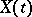on some intervalusing its observed values outside this interval. Usually one has in mind the interpolation estimator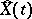for which the mean-square error of interpolation is minimal compared to all other estimators:the interpolation is called linear if one restricts attention to linear estimators. One of the first problems posed and solved was that of linear interpolation of the value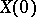of a stationary sequence. This problem is analogous to the following one: In the space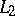of square-integrable functions on the interval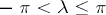, one must find the projection of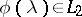onto the subspace generated by the functions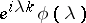,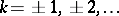. This problem has been greatly generalized in the theory of stationary stochastic processes (cf. Stationary stochastic process; , ). One application is the problem of interpolation of the stochastic process arising from the system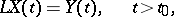whereis a linear differential operator of order, and,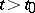, is a white noise process. For given initial values,, independent of the white noise, the optimal interpolation estimator,, is the solution of the corresponding boundary value problemwhere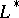denotes the formal adjoint operator,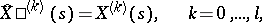with boundary conditions at the boundary points. For systems of stochastic differential equations the problem of interpolation of some components given the values of other observed components reduces to similar interpolation equations. (See .)

How to Cite This Entry:
Stochastic processes, interpolation of. Encyclopedia of Mathematics. URL: http://encyclopediaofmath.org/index.php?title=Stochastic_processes,_interpolation_of&oldid=17488
This article was adapted from an original article by Yu.A. Rozanov (originator), which appeared in Encyclopedia of Mathematics - ISBN 1402006098. See original article